# Bench-to-bedside review: Fundamental principles of acid-base physiology

## Abstract

Complex acid–base disorders arise frequently in critically ill patients, especially in those with multiorgan failure. In order to diagnose and treat these disorders better, some intensivists have abandoned traditional theories in favor of revisionist models of acid–base balance. With claimed superiority over the traditional approach, the new methods have rekindled debate over the fundmental principles of acid–base physiology. In order to shed light on this controversy, we review the derivation and application of new models of acid–base balance.

## Introduction: Master equations

All modern theories of acid–base balance in plasma are predicated upon thermodynamic equilibrium equations. In an equilibrium theory, one enumerates some property of a system (such as electrical charge, proton number, or proton acceptor sites) and then distributes that property among the various species of the system according to the energetics of that particular system. For example, human plasma consists of fully dissociated ions ('strong ions' such as Na+, K+, Cl-and lactate), partially dissociated 'weak' acids (such as albumin and phosphate), and volatile buffers (carbonate species). CB, the total concentration of proton acceptor sites in solution, is given by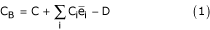Where C is the total concentration of carbonate species proton acceptor sites (in mmol/l), Ci is the concentration of noncarbonate buffer species i (in mmol/l),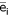is the average number of proton acceptor sites per molecule of species i, and D is Ricci's difference function (D = [H+] - [OH-]). Equation 1 may be regarded as a master equation from which all other acid–base formulae may be derived .

Assuming that [CO32-] is small, Eqn 1 may be re-expressed: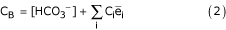Similarly, the distribution of electrical charge may be expressed as follows: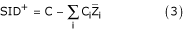Where SID+ is the 'strong ion difference' and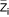is the average charge per molecule of species i.

The solution(s) to these master equations require rigorous mathematical modeling of complex protein structures. Traditionally, the mathematical complexity of master Eqn 2 has been avoided by setting ΔCi = 0, so that ΔCB = Δ[HCO3-]. The study of acid–base balance now becomes appreciably easier, simplifying essentially to the study of volatile buffer equilibria.

### Stewart equations

Stewart, a Canadian physiologist, held that this simplification is not only unnecessary but also potentially misleading [2, 3]. In 1981, he proposed a novel theory of acid–base balance based principally on an explicit restatement of master Eqn 3:

Bicarbonate ion formation equilibrium:

[H+] × [HCO3-] = K'1 × S × PCO2     (4)

Where K'1 is the apparent equilibrium constant for the Henderson–Hasselbalch equation and S is the solubility of CO2 in plasma.

Carbonate ion formation equilibrium:

[H+] × [CO3-2] = K3 × [HCO3-]     (5)

Where K3 is the apparent equilibrium dissociation constant for bicarbonate.

Water dissociation equilibrium:

[H+] × [OH-] = K'w     (6)

Where K'w is the autoionization constant for water.

Electrical charge equation:

[SID+] = [HCO3-] + [A-] + [CO3-2] + [OH-] - [H+]     (7)

Where [SID+] is the difference in strong ions ([Na+] + [K+] - [Cl-] - [lactate-]) and [A-] is the concentration of dissociated weak acids, mostly albumin and phosphate.

Weak acid dissociation equilibrium:

[H+] × [A-] = Ka × [HA]     (8)

Where Ka is the weak acid dissociation constant for HA.

In addition to these five equations based principally on the conservation of electrical charge, Stewart included one additional equation.

Conservation of mass for 'A':

[ATOT] = [HA] + [A-]     (9)

Where [ATOT] is the total concentration of weak acids.

Accordingly, [H+] may be determined only if the constraints of all six of the equations are satisfied simultaneously [2, 3]. Combining equations, we obtain:

a [H+]4 + b [H+]3 + c [H+]2 + d [H+] + e = 0     (10)

Where a = 1; b = [SID+] + Ka; c = {Ka × ([SID+] - [ATOT]) -

K'w - K'1 × S × PCO2}; d = -{Ka × (K'w + K'1 × S × PCO2) -

K3 × K'1 × S × PCO2}; and e = -KaK3K'1S PCO2.

If we ignore the contribution of the smaller terms in the electrical charge equation (Eqn 7), then Eqn 10 simplifies to become :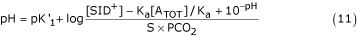In traditional acid–base physiology, [ATOT] is set equal to 0 and Eqn 11 is reduced to the well-known Henderson–Hasselbalch equation [5, 6]. If this simplification were valid, then the plot of pH versus log PCO2 ('the buffer curve') would be linear, with an intercept equal to log [HCO3-]/K'1 × SCO2 [7, 8]. In fact, experimental data cannot be fitted to a linear buffer curve . As indicated by Eqn 11, the plot of pH versus log PCO2 is displaced by changes in protein concentration or the addition of Na+ or Cl-, and becomes nonlinear in markedly acid plasma (Fig. 1). These observations suggest that the Henderson–Hasselbalch equation may be viewed as a limiting case of the more general Stewart equation. When [ATOT] varies, the simplifications of the traditional acid–base model may be unwarranted .

### The Stewart variables

The Stewart equation (Eqn 10) is a fourth-order polynomial equation that relates [H+] to three independent variables ([SID+], [ATOT] and PCO2) and five rate constants (Ka, K'w, K'1, K3 and SCO2), which in turn depend on temperature and ion activities (Fig. 2) [2, 3].

#### Strong ion difference

The first of these three variables, [SID+], can best be appreciated by referring to a 'Gamblegram' (Fig. 3). The 'apparent' strong ion difference, [SID+]a, is given by the following equation:

[SID+]a = [Na+] + [K+] - [Cl-] - [lactate] - [other strong anions]     (12)

In normal plasma, [SID+]a is equal to [SID+]e, the 'effective' strong ion difference:

[SID+]e = [HCO3-] + [A-]     (13)

Where [A-] is the concentration of dissociated weak noncarbonic acids, principally albumin and phosphate.

#### Strong ion gap

The strong ion gap (SIG), the difference between [SID+]a and [SID+]e, may be taken as an estimate of unmeasured ions:

SIG = [SID+]a - [SID+]e= AG - [A-]     (14)

Unlike the well-known anion gap (AG = [Na+] + [K+] - [Cl-] - [HCO3-]) , the SIG is normally equal to 0.

SIG may be a better indicator of unmeasured anions than the AG. In plasma with low serum albumin, the SIG may be high (reflecting unmeasured anions), even with a completely normal AG. In this physiologic state, the alkalinizing effect of hypoalbuminemia may mask the presence of unmeasured anions .

#### Weak acid buffers

Stewart defined the second variable, [ATOT], as the composite concentration of the weak acid buffers having a single dissociation constant (KA = 3.0 × 10-7) and a net maximal negative charge of 19 mEq/l [2, 3]. Because Eqn 9 invokes the conservation of mass and not the conservation of charge, Constable  computed [ATOT] in units of mass (mmol/l) rather than in units of charge (mEq/l), and found that [ATOT (mmol/l)] = 5.72 ± 0.72 [albumin (g/dl)].

Although thermodynamic equilibrium equations are independent of mechanism, Stewart asserted that his three independent parameters ([SID+], [ATOT] and PCO2) determine the only path by which changes in pH may arise (Fig. 4). Furthermore, he claimed that [SID+], [ATOT] and PCO2 are true biologic variables that are regulated physiologically through the processes of transepithelial transport, ventilation, and metabolism (Fig. 5).

#### Base excess

In contrast to [SID+], the 'traditional' parameter base excess (BE; defined as the number of milliequivalents of acid or base that are needed to titrate 1 l blood to pH 7.40 at 37°C while the PCO2 is held constant at 40 mmHg) provides no further insight into the underlying mechanism of acid–base disturbances [20, 21]. Although BE is equal to ΔSID+when nonvolatile buffers are held constant, BE is not equal to ΔSID+ when nonvolatile acids vary. BE read from a standard nomogram is then not only physiologically unrevealing but also numerically inaccurate (Fig. 2) [1, 9].

#### The Stewart theory: summary

The relative importance of each of the Stewart variables in the overall regulation of pH can be appreciated by referring to a 'spider plot' (Fig. 6). pH varies markedly with small changes in PCO2 and [SID+]. However, pH is less affected by perturbations in [ATOT] and the various rate constants .

In summary, in exchange for mathematical complexity the Stewart theory offers an explanation for anomalies in the buffer curve, BE, and AG.

### The Figge–Fencl equations

Based on the conservation of mass rather than conservation of charge, Stewart's [ATOT] is the composite concentration of weak acid buffers, mainly albumin. However, albumin does not exhibit the chemistry described by Eqn 9 within the range of physiologic pH, and so a single, neutral [AH] does not actually exist . Rather, albumin is a complex polyampholyte consisting of about 212 amino acids, each of which has the potential to react with [H+].

From electrolyte solutions that contained albumin as the sole protein moiety, Figge and coworkers [23, 24] computed the individual charges of each of albumin's constituent amino acid groups along with their individual pKa values. In the Figge–Fencl model, Stewart's [ATOT] term is replaced by [Pix-] and [Pry-] (the contribution of phosphate and albumin to charge balance, respectively), so that the four independent variables of the model are [SID+], PCO2, [Pix-], and [Pry-].

Omitting the small terms

[SID+] - [HCO3-] - [Pix-] - [Pry-] = 0     (15)

The Figge–Fencl equation is as follows :

SID+ + 1000 × ([H+] - Kw/ [H+] - Kc1 × PCO2/

[H+] - Kc1 × Kc2 × PCO2/ [H+]2) - [Pitot] × Z

+ {-1/(1 + 10- [pH-8.5])

98/(1 + 10- [pH-4.0])

18/(1 + 10- [pH-10.9])

+ 24/(1 + 10+ [pH-12.5])

+ 6/(1 + 10+ [pH-7.8])

+ 53/(1 + 10+ [pH-10.0])

+ 1/(1 + 10+ [pH-7.12+NB])

+ 1/(1 + 10+ [pH-7.22+NB])

+ 1/(1 + 10+ [pH-7.10+NB])

+ 1/(1 + 10+ [pH-7.49+NB])

+ 1/(1 + 10+ [pH-7.01+NB])

+ 1/(1 + 10+ [pH-7.31])

+ 1/(1 + 10+ [pH-6.75])

+ 1/(1 + 10+ [pH-6.36])

+ 1/(1 + 10+ [pH-4.85])

+ 1/(1 + 10+ [pH-5.76])

+ 1/(1 + 10+ [pH-6.17])

+ 1/(1 + 10+ [pH-6.73])

+ 1/(1 + 10+ [pH-5.82])

+ 1/(1 + 10+ [pH-6.70])

+ 1/(1 + 10+ [pH-4.85])

+ 1/(1 + 10+ [pH-6.00])

+ 1/(1 + 10+ [pH-8.0])

1/(1 + 10- [pH-3.1])} × 1000 × 10 × [Alb]/66500 = 0     (16)

Where [H+] = 10-pH; Z = (K1 × [H+]2 + 2 × K1 × K2 × [H+] + 3 × K1 × K2 × K3)/([H+]3 + K1 × [H+]2 + K1 × K2 × [H+] + K1 × K2 × K3); and NB = 0.4 × (1 - 1/(1 + 10 [pH-6.9])).

The strong ion difference [SID+] is given in mEq/l, PCO2 is given in torr, the total concentration of inorganic phosphorus containing species [Pitot] is given in mmol/l and [Alb] is given in g/dl. The various equilibrium constants are Kw = 4.4 × 10-14 (Eq/l)2; Kc1 = 2.46 × 10-11 (Eq/l)2/torr; Kc2 = 6.0 × 10-11 (Eq/l); K1 = 1.22 × 10-2 (mol/l); K2 = 2.19 × 10-7 (mol/l); and K3 = 1.66 × 10-12(mol/l).

Watson  has provided a simple way to understand the Figge–Fencl equation. In the pH range 6.8–7.8, the pKa values of about 178 of the amino acids are far from the normal pH of 7.4. As a result, about 99 amino acids will have a fixed negative charge (mainly aspartic acid and glutamic acid) and about 79 amino acids will have a fixed positive charge (mostly lysine and arginine), for a net fixed negative charge of about 21 mEq/mol. In addition to the fixed charges, albumin contains 16 histidine residues whose imidazole groups may react with H+ (variable charges).

The contribution of albumin to charge, [Prx-], can then be determined as follows:

[Prx-] = 21- (16 × [1 - αpH]) × 10,000/66,500 × [albumin (g/dl)]     (17)

Where 21 is the number of 'fixed' negative charges/mol albumin, 16 is the number of histidine residues/mol albumin, and αpH is the ratio of unprotonated to total histadine at a given pH. Equation Equ17 yields identical results to the more complex Figge–Fencl analysis.

#### Linear approximations

In the linear approximation taken over the physiologic range of pH, Eqn 16 becomes

[SID+]e = [HCO3-] + [PrX-] + [PiY-]     (18)

Where [HCO3-] = 1000 × Kcl × PCO2/(10-pH); [PrX-] = [albumin (g/dl)] (1.2 × pH-6.15) is the contribution of albumin to charge balance; and [PiY-] = [phosphate (mg/dl)] (0.097 × pH-0.13) is contribution of phosphate to charge balance [1, 2325].

Combining equations yields the following:

SIG = AG - [albumin (g/dl)] (1.2 × pH-6.15) - [phosphate (mg/dl)] (0.097 × pH-0.13)     (19)

According to Eqn 18, when pH = 7.40 the AG increases by roughly 2.5 mEq/l for every 1 g/dl decrease in [albumin].

#### Buffer value

The buffer value (β) of plasma, defined as β = Δbase/ΔpH, is equal to the slope of the line generated by plotting (from Eqn 18) [SID+]e versus pH :

β = 1.2 × [albumin (g/dl)] + 0.097 × [phosphate (mg/dl)]     (20)

When plasma β is low, the ΔpH is higher for any given BE than when β is normal.

The β may be regarded as a central parameter that relates the various components of the Henderson–Hasselbalch, Stewart and Figge–Fencl models together (Fig. 7). When non-carbonate buffers are held constant:

BE = Δ[SID+]e= Δ[HCO3-] + βΔpH     (21)

When non-carbonate buffers vary, BE = Δ[SID+]e'; that is, [SID+]a referenced to the new weak buffer concentration.

#### The Figge–Fencl equations: summary

In summary, the Figge–Fencl model relates the traditional to the Stewart parameters and provides equations that permit β, [SID+]e, and SIG to be calculated from standard laboratory measurements.

### The Wooten equations

Acid–base disorders are usually analyzed in plasma. However, it has long been recognized that the addition of hemoglobin [Hgb], an intracellular buffer, to plasma causes a shift in the buffer curve (Fig. 8) . Therefore, BE is often corrected for [Hgb] using a standard nomogram [20, 21, 27].

Wooten  developed a multicompartmental model that 'corrects' the Figge–Fencl equations for [Hgb]:

β = (1 - Hct) 1.2 × [albumin (g/dl)] + (1 - Hct) 0.097 × [phosphate (mg/dl)] + 1.58 [Hgb (g/dl)] + 4.2 (Hct)     (22)

[SID+]effective, blood = (1 - 0.49 × Hct) [HCO3-] +

(1 - Hct)(Calb [1.2 × pH-6.15] + Cphos [0.097 ×

pH-0.13]) + CHgb (1.58 × pH-11.4) + Hct (4.2 × pH-3.3)     (23)

With Calb and CHgb expressed in g/dl and Cphos in mg/dl.

In summary, the Wooten model brings Stewart theory to the analysis of whole blood and quantitatively to the level of titrated BE.

## Application of new models of acid–base balance

In order to facilitate the implementation of the Stewart approach at the bedside, Watson  has developed a computer program (AcidBasics II) with a graphical user interface (Fig. 9). One may choose to use the original Stewart or the Figge–Fencl model, vary any of the rate constants, or adjust the temperature. Following the input of the independent variables, the program automatically displays all of the independent variables, including pH, [HCO3-] and [A-]. In addition, the program displays SIG, BE, and a 'Gamblegram' (for an example, see Fig. 3).

One may classify acid–based disorders according to Stewart's three independent variables. Instead of four main acid–base disorders (metabolic acidosis, metabolic alkalosis, respiratory acidosis, and respiratory alkalosis), there are six disorders based on consideration of PCO2, [SID+], and [ATOT] (Table 1). Disease processes that may be diagnosed using the Stewart approach are listed in Table 2.

### Example

Normal plasma may be defined by the following values: pH = 7.40, PCO2 = 40.0 torr, [HCO3-] = 24.25 mmol/l, [albumin] = 4.4 g/dl, phosphate = 4.3 mg/dl, sodium = 140 mEq/l, potassium = 4 mEq/l, and chloride = 105 mEq/l. The corresponding values for 'traditional' and 'Stewart' acid–base parameters are listed in Table 3.

Consider a hypothetical 'case 1' with pH = 7.30, PCO2 = 30.0 torr, [HCO3-] = 14.25 mmol/l, Na2+ = 140 mEq/l, K+ = 4 mEq/l, Cl- = 115 mEq/l, and BE = -10 mEq/l. The 'traditional' interpretation based on BE and AG is a 'normal anion gap metabolic acidosis' with respiratory compensation. The Stewart interpretation based on [SID+]e and SIG is 'low [SID+]e/normal SIG' metabolic acidosis and respiratory compensation. The Stewart approach 'corrects' the BE read from a nomogram for the 0.6 mEq/l acid load 'absorbed' by the noncarbonate buffers. In both models, the differential diagnosis for the acidosis includes renal tubular acidosis, diarrhea losses, pancreatic fluid losses, anion exchange resins, and total parenteral nutrition (Tables 2 and 3).

Now consider a hypothetical 'case 2' with the same arterial blood gas and chemistries but with [albumin] = 1.5 g/dl. The 'traditional' interpretation and differential diagnosis of the disorder remains unchanged from 'case 1' because BE and AG have not changed. However, the Stewart interpretation is low [SID+]e/high SIG metabolic acidosis and respiratory compensation. Because of the low β, the ΔpH is greater for any given BE than in 'case 1'. The Stewart approach corrects BE read from a nomogram for the 0.2 mEq/l acid load 'absorbed' by the noncarbonate buffers. The differential diagnosis for the acidosis includes ketoacidosis, lactic acidosis, salicylate intoxication, formate intoxication, and methanol ingestion (Tables 2 and 3).

## Summary

All modern theories of acid–base balance are based on physiochemical principles. As thermodynamic state equations are independent of path, any convenient set of parameters (not only the one [s] used by nature) may be used to describe a physiochemical system. The traditional model of acid–base balance in plasma is based on the distribution of proton acceptor sites (Eqn 1), whereas the Stewart model is based on the distribution of electrical charge (Eqn 2). Although sophisticated and mathematically equivalent models may be derived from either set of parameters, proponents of the 'traditional' or 'proton acceptor site' approach have advocated simple formulae whereas proponents of the Stewart 'electrical charge' method have emphasized mathematical rigor.

The Stewart model examines the relationship between the movement of ions across biologic membranes and the consequent changes in pH. The Stewart equation relates changes in pH to changes in three variables, [SID+], [ATOT] and PCO2. These variables may define a biologic system and so may be used to explain any acid–base derangement in that system.

Figge and Fencl further refined the model by analyzing explicitly each of the charged residues of albumin, the main component of [ATOT]. Wooten extended these observations to multiple compartments, permitting the consideration of both extracellular and intracellular buffers.

In return for mathematical complexity, the Stewart model 'corrects' the 'traditional' computations of buffer curve, BE, and AG for nonvolative buffer concentration. This may be important in critically ill, hypoproteinuric patients.

## Conclusion

Critics note that nonvolatile buffers contribute relatively little to BE and that a 'corrected' AG (providing similar information to the SIG) may be calculated without reference to Stewart theory by adding about 2.5 × (4.4 - [albumin]) to the AG.

To counter these and other criticisms, future studies need to demonstrate the following: the validity of Stewart's claim that his unorthodox parameters are the sole determinants of pH in plasma; the prognostic significance of the Stewart variables; the superiority of the Stewart parameters for patient management; and the concordance of the Stewart equations with experimental data obtained from ion transporting epithelia.

In the future, the Stewart model may be improved through a better description of the electrostatic interaction of ions and polyelectroles (Poisson–Boltzman interactions). Such interactions are likely to have an important effect on the electrical charges of the nonvolatile buffers. For example, a detailed analysis of the pH-dependent interaction of albumin with lipids, hormones, drugs, and calcium may permit further refinement of the Figge–Fencl equation .

Perhaps most importantly, the Stewart theory has reawakened interest in quantitative acid–base chemistry and has prompted a return to first principles of acid–base physiology.

## Abbreviations

AG:

anion gap

[ATOT]:

= total concentration of weak acids

BE:

= base excess

PCO2:

= partial CO2 difference

SCO2:

= CO2 solubility

SID+:

= strong ion difference

SIG:

= strong ion gap.

## References

1. Wooten EW: Analytic calculation of physiological acid–base parameters. J Appl Physiol 1999, 86: 326-334.

2. Stewart PA: How to understand acid base balance. In In A Quantitative Acid–Base Primer for Biology and Medicine. New York: Elsevier; 1981.

3. Stewart PA: Modern quantitative acid-base chemistry. Can J Physiol Pharmacol 1983, 61: 1444-1461.

4. Constable PD: A simplified strong ion model for acid-base equilibria: application to horse plasma. J Appl Physiol 1997, 83: 297-311.

5. Hasselbalch KA, Gammeltoft A: The neutral regulation of the gravid organism [in German]. Biochem Z 1915, 68: 206.

6. Hasselbalch KA: The 'reduced' and the 'regulated' hydrogen number of the blood [in German]. Biochem Z 1918, 174: 56.

7. Van Slyke DD: Studies of acidosis: XVII. The normal and abnormal variations in the acid base balance of the blood. J Biol Chem 1921, 48: 153.

8. Siggaard-Andersen O: The pH-log PCO 2 blood acid–base nomogram revised. Scand J Clin Lab Invest 1962, 14: 598-604.

9. Corey HE: Stewart and beyond: new models of acid-base balance. Kidney Int 2003, 64: 777-787. 10.1046/j.1523-1755.2003.00177.x

10. Oh MS, Carroll HJ: Current concepts: the anion gap. N Engl J Med 1977, 297: 814.

11. Constable PD: Clinical assessment of acid–base status. Strong ion difference theory. Vet Clin North Am Food Anim Pract 1999, 15: 447-472.

12. Fencl V, Jabor A, Kazda A, Figge J: Diagnosis of metabolic acid-base disturbances in critically ill patients. Am J Respir Crit Care Med 2000, 162: 2246-2251.

13. Jurado RL, Del Rio C, Nassar G, Navarette J, Pimentel JL Jr: Low anion gap. South Med J 1998, 91: 624-629.

14. McAuliffe JJ, Lind LJ, Leith DE, Fencl V: Hypoproteinemic alkalosis. Am J Med 1986, 81: 86-90. 10.1016/0002-9343(86)90187-7

15. Rossing TH, Maffeo N, Fencl V: Acid–base effects of altering plasma protein concentration in human blood in vitro. J Appl Physiol 1986, 61: 2260-2265.

16. Constable PD, Hinchcliff KW, Muir WW: Comparison of anion gap and strong ion gap as predictors of unmeasured strong ion concentration in plasma and serum from horses. Am J Vet Res 1998, 59: 881-887.

17. Kellum JA, Kramer DJ, Pinsky MR: Strong ion gap: a methodology for exploring unexplained anions. J Crit Care 1995, 10: 51-55. 10.1016/0883-9441(95)90016-0

18. Figge J, Jabor A, Kazda A, Fencl V: Anion gap and hypoalbuminemia. Crit Care Med 1998, 26: 1807-1810.

19. Constable PD: Total weak acid concentration and effective dissociation constant of nonvolatile buffers in human plasma. J Appl Physiol 2001, 91: 1364-1371.

20. Siggaard-Andersen O, Engel K: A new acid–base nomogram, an improved method for calculation of the relevant blood acid–base data. Scand J Clin Lab Invest 1960, 12: 177.

21. Siggaard-Andersen O: Blood acid–base alignment nomogram. Scales for pH, PCO 2 , base excess of whole blood of different hemoglobin concentrations, plasma bicarbonate and plasma total CO 2 . Scand J Clin Lab Invest 1963, 15: 211-217.

22. Watson PD: Modeling the effects of proteins on pH in plasma. J Appl Physiol 1999, 86: 1421-1427.

23. Figge J, Rossing TH, Fencl V: The role of serum proteins in acid–base equilibria. J Lab Clin Med 1991, 117: 453-467.

24. Figge J, Mydosh T, Fencl V: Serum proteins and acid-base equilibria: a follow-up. J Lab Clin Med 1992, 120: 713-719.

25. Figge J: An Educational Web Site about Modern Human Acid-Base Physiology: Quantitative Physicochemical Model.[http://www.figgefencl.org]

26. Davenport HW: The ABC of Acid–Base Chemistry. Chicago: University of Chicago Press; 1974.

27. Singer RB, Hastings AB: Improved clinical method for estimation of disturbances of acid-base balance of human blood. Medicine 1948, 27: 223-242.

28. Wooten EW: Calculation of physiological acid–base parameters in multicompartment systems with application to human blood. J Appl Physiol 2003, 95: 2333-2344.

29. Watson PD: USC physiology acid–base center: software and data sets.[http://www.med.sc.edu:96/watson/Acidbase/Acidbase.htm]

## Acknowledgments

I would like to acknowledge the helpful discussions I have had with Dr E Wrenn Wooten and Dr P Watson during the preparation of the manuscript.

## Author information

Authors

### Corresponding author

Correspondence to Howard E Corey.

### Competing interests

The author(s) declare that they have no competing interests.

## Authors’ original submitted files for images

Below are the links to the authors’ original submitted files for images.

## Rights and permissions

Reprints and Permissions

Corey, H.E. Bench-to-bedside review: Fundamental principles of acid-base physiology. Crit Care 9, 184 (2004). https://doi.org/10.1186/cc2985